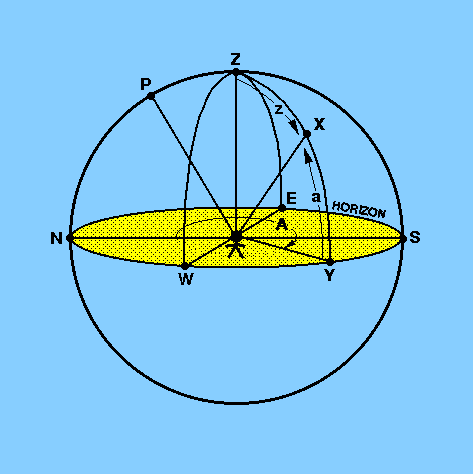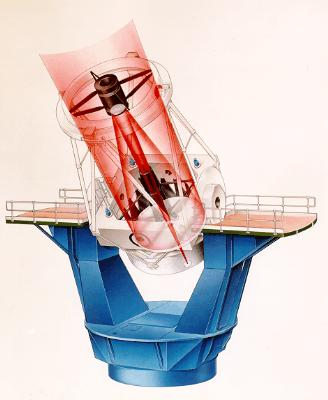The horizontal coordinate system (commonly referred to as the alt-az system) is the simplest coordinate system as it is based on the observer's horizon. The celestial hemisphere viewed by an observer on the Earth is shown in Figure 13. The great circle through the zenith Z and the north celestial pole P cuts the horizon NESYW at the north point (N) and the south point (S). The great circle WZE at right angles to the great circle NPZS cuts the horizon at the west point (W) and the east point (E). The arcs ZN, ZW, ZY, etc, are known as verticals.

 figure 13: The horizontal coordinate system.The two numbers which specify the position of a star, X, in this system are the azimuth, A, and the altitude, a. The altitude of X is the angle measured along the vertical through X from the horizon at Y to X. It is measured in degrees. An often-used alternative to altitude is the zenith distance, z, of X, indicated by ZX in Figure 13. Clearly, z = 90 - a. Azimuth may be defined in a number of ways. For the purposes of this course, azimuth will be defined as the angle between the vertical through the north point and the vertical through the star at X, measured eastwards from the north point along the horizon from 0° to 360°. This definition applies to observers in both the northern and the southern hemispheres.

It is often useful to know how high a star is above the horizon and in what direction it can be found - this is the main advantage of the alt-az system. The main disadvantage of the alt-az system is that it is a local coordinate system - i.e. two observers at different points on the Earth's surface will measure different altitudes and azimuths for the same star at the same time. In addition, an observer will find that the star's alt-az coordinates changes with time as the celestial sphere appears to rotate. Despite these problems, most modern research telescopes use alt-az mounts, as shown in Figure 14, owing to their lower cost and greater stability. This means that computer control systems which can transform alt-az coordinates to equatorial coordinates are required. An example of such a coordinate transformation is given in the example problems.

 figure 14: An alt-az mounted telescope - the 4.2 m William Herschel Telescope (WHT) on La Palma.